﻿ 题解 UVA1073 【Glenbow Museum】 – Hzao's Blog

# 题解 UVA1073 【Glenbow Museum】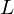个内角代表条边，根据多边形内角和公式：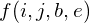表示有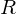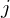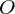，开头为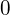表示表示），结尾为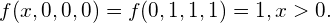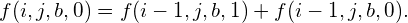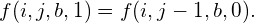（不能出现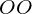``````#include<cstdio>
#include<cstring>
int L,kase;
long long d;
long long f(int i,int j,int b,int e){
if(~d[i][j][b][e]){
return d[i][j][b][e];
}
if(!j){
return i&&!b&&!e;
}
if(!i){
return j==1&&b==1&&e==1;
}
if(e){
return d[i][j][b][e]=f(i,j-1,b,0);
}
else{
return d[i][j][b][e]=f(i-1,j,b,0)+f(i-1,j,b,1);
}
}
int main(){
memset(d,-1,sizeof(d));
long long ans;
while(~scanf("%d",&L)&&L){
ans=0;
if(!(L&1||L<4)){
ans+=f((L+4)/2,(L-4)/2,0,0);
ans+=f((L+4)/2,(L-4)/2,1,0);
ans+=f((L+4)/2,(L-4)/2,0,1);
}
printf("Case %d: %lld\n",++kase,ans);
}
return 0;
}``````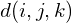表示以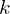开头，有，有对相邻的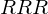算两对，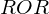对），且以结尾的方案数。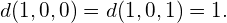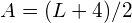``````#include<cstdio>
#include<cstring>
int L,kase;
long long d;
long long f(int i,int j,int k){
if(i==1){
return !j;
}
if(~d[i][j][k])return d[i][j][k];
return d[i][j][k]=f(i-1,j,k)+f(i-1,j-1,k);
}
int main(){
memset(d,-1,sizeof(d));
while(~scanf("%d",&L)&&L){
if(L&1||L<4){
printf("Case %d: 0\n",++kase);
continue;
}
printf("Case %d: %lld\n",++kase,
f((L+4)/2,3,0)+//R...R
f((L+4)/2,4,1)+//O...R
f((L+4)/2,4,0)  //R...O
);
}
return 0;
}``````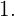在开头放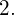不在开头放